# SDI-12

• Has anyone written or know of an SDI-12 library for Micropython or the Pycom board.

Specifically I need to interface to the Decagon 5TM sensor from the LoPy. Any suggestions?

• Sorry to grave dig, but had I known this earlier it would have been helpful. This post is about the Decagon 5TM, which has two protocols, SDI-12 and DDI. The DDI is typical UART at 1200 baud, which you can read from any UART reader. I've tested it with an FTDI cable and know it works with a LoPy. You do however have to power cycle the sensor to get a new reading.

Note: This may not work on new Decagon 5TM sensors if you have changed the SDI-12 address to anything other than 0. Factory default is 0. If you have not used SDI-12 to communicate with the sensor then it should work as expected.

We used a mosfet to turn the power on and off for the sensor, controlled by GPIO. Reading the UART within 10s of milliseconds from turning the power on will give you your readings. Its all explained in the integration guide.

http://publications.metergroup.com/Integration Guides/18237 5TM Integrators Guide.pdf

I know this doesn't help with SDI-12, but jubbs is using the 5TM specifically, which has this dual protocol stack.

The sensor was also just powered from 5 volts (same supply source as for the LoPy), the 5TM supports 3.6V-15V, check datasheet for more information including current draw.

• @robert-hh

ok changed cct to replicate below and am starting to get response from sensor, however thinking their is something wrong with the timings in the "SDI12(cmd)".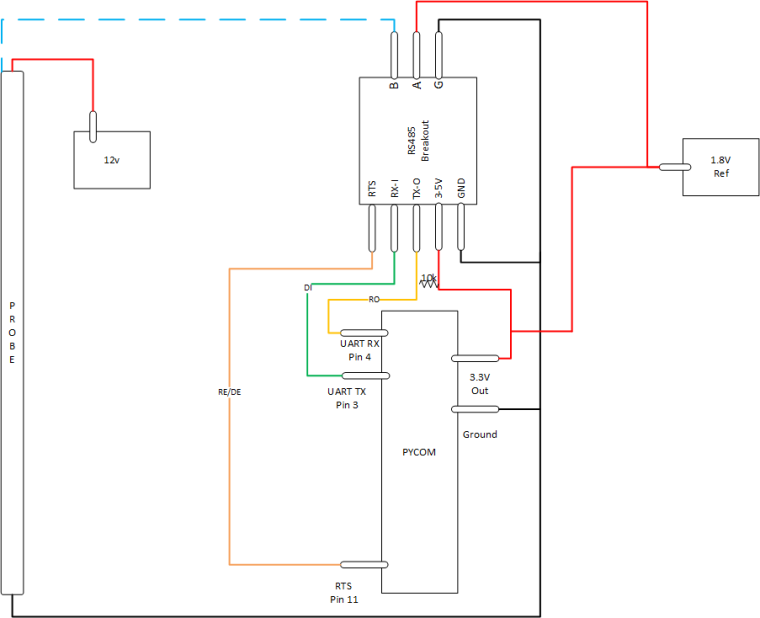The following code gets me the below responses the first 3 are as expected, the last one I'm expecting 12 temperature readings separated by "+0".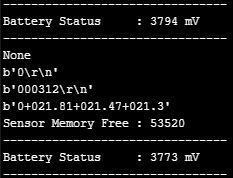``````import network
import time
import pycom
import machine
import gc
import socket
import utime
from machine import RTC
from machine import Pin
from machine import UART
from network import LoRa

execfile('/flash/Network/LoRa.py')

f_device = open('/flash/Config/Device_Details.txt', 'r')

device_strings = [i.strip() for i in device_setting.split(',')]
f_device.close()

pycom.heartbeat(False)
rtc = RTC()
lora = LoRa()
uart = UART(1, baudrate=1200)
p_tx = Pin('P3', mode=Pin.OUT)
p_dir = Pin('P11', mode=Pin.OUT)

def SDI12(cmd):
uart.deinit()
p_tx.value(0)
p_dir.value(1)                                                          # set output dir
utime.sleep_us(12500)                                                   # send BREAK
p_tx.value(1)
utime.sleep_us(8333)                                                    # send MARK
uart.init(1200, bits=7, parity=UART.EVEN, stop=1, timeout_chars=75)     # init with given parameters
uart.write(cmd)                                                         # send command
utime.sleep_us(8333 * len(cmd))                                               # wait to send command (byte time * command lenght)
p_dir.value(0)                                                          # output set to read
return line;

time.sleep(2)

while True:
#s = socket.socket(socket.AF_LORA, socket.SOCK_RAW)
#s.setsockopt(socket.SOL_LORA, socket.SO_DR, 5)
#s.setblocking(True)
#s.send("Battery Status : " + str(batt.value()) + " mV")
#s.setblocking(False)
print('--------------------------------')
print("Battery Status     : " + str(batt.value()) + " mV")
print('--------------------------------')
##########################################################
#                   Measure Temperature                  #
##########################################################
utime.sleep(2)
wake_up = SDI12('?!')           #Wake Up Sensor
print(wake_up)                  #Print Sensor Response
utime.sleep(2)                  #Wait before Next Cmd
utime.sleep(2)                  #Wait before Next Cmd
temp_read = SDI12('0C2!')       #Probe Temp Measure
utime.sleep(2)                  #Wait before Next Cmd
temp_data = SDI12('0D0!')       #Probe Temp Readings
##########################################################
#                     Garbage Collect                    #
##########################################################
gc.enable()
gc.collect()
print("Sensor Memory Free : " + str(gc.mem_free()))
time.sleep(15)`````````

• @jimpower You need alsol to connect A of the breakout board to a reference potential, like 1.8 V, as shown in the initial drawing. You must not leave it open, otherwise there will be not signal current.

• @jimpower I think you have to connect the 12V ground to the Pycom ground.
Can you send me the RS485 Breakout reference?

• @antoniovalente I have tried to implement your code with a different SDI-12 probe I am however getting a "none" response .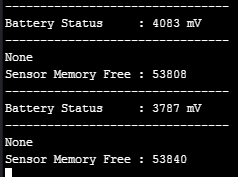Here is my connections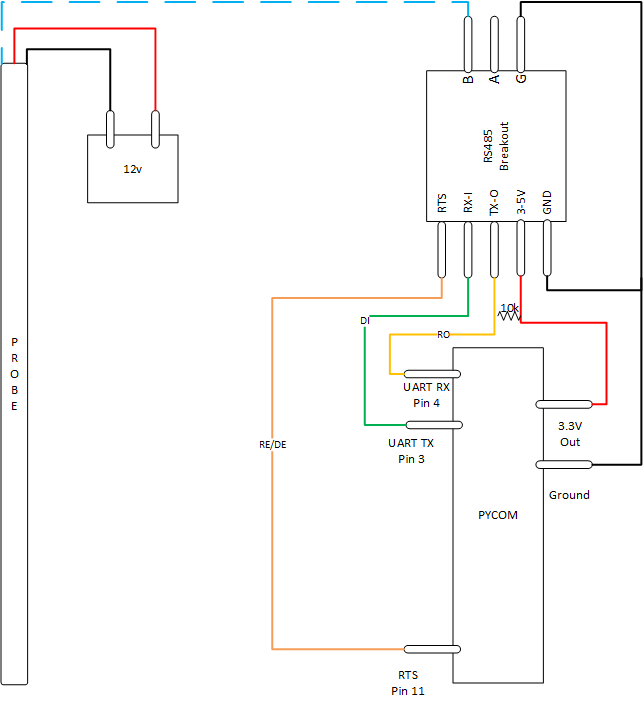``````import network
import time
import pycom
import machine
import gc
import socket
import utime
from machine import RTC
from machine import Pin
from machine import UART
from network import LoRa

execfile('/flash/Network/LoRa.py')

f_device = open('/flash/Config/Device_Details.txt', 'r')

device_strings = [i.strip() for i in device_setting.split(',')]
f_device.close()

pycom.heartbeat(False)
rtc = RTC()
lora = LoRa()
uart = UART(1, baudrate=1200)
p_tx = Pin('P3', mode=Pin.OUT)
p_dir = Pin('P11', mode=Pin.OUT)

def SDI12(cmd):
uart.deinit()
p_tx.value(0)
p_dir.value(1)                                                          # set output dir
utime.sleep_us(25000)                                                   # send BREAK
p_tx.value(1)
utime.sleep_us(8333)                                                    # send MARK
uart.init(1200, bits=7, parity=UART.EVEN, stop=1, timeout_chars=75)     # init with given parameters
uart.write(cmd)                                                         # send command
utime.sleep_us(8333 * len(cmd))                                         # wait to send command (byte time * command lenght)
p_dir.value(0)                                                          # output set to read
return line;

time.sleep(2)

while True:
#s = socket.socket(socket.AF_LORA, socket.SOCK_RAW)
#s.setsockopt(socket.SOL_LORA, socket.SO_DR, 5)
#s.setblocking(True)
#s.send("Battery Status : " + str(batt.value()) + " mV")
#s.setblocking(False)
print('--------------------------------')
print("Battery Status     : " + str(batt.value()) + " mV")
print('--------------------------------')
##########################################################
#                   Measure Temperature                  #
##########################################################
#line = ('000312')
utime.sleep(2)
line = SDI12('?!')
print(line)
#temp_time_samp = int(line[1:4])
#temp_sens_samp = int(line[4:6])
#print("Temp Read Time     : " + str(temp_time_samp) + " Secs")
#print("Temp Sensor Read   : " + str(temp_sens_samp) + " Sensors")
##########################################################
#                     Garbage Collect                    #
##########################################################
gc.enable()
gc.collect()
print("Sensor Memory Free : " + str(gc.mem_free()))
time.sleep(10)`````````

• @senseit The pin (P11) is for selecting between send and receive. It is connected to ~RE and DE and in the code is p_dir.

``````p_tx   = Pin('P3' , mode=Pin.OUT)
p_dir   = Pin('P11', mode=Pin.OUT)
``````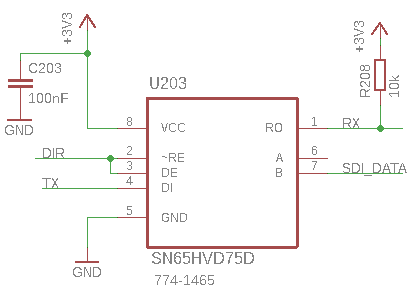The pinout of SN65HVD75 is the same of SN65HVD1780.

• @antoniovalente Thank you for the instructions and code. Could you please indicate where in the code the data pin (P11) is specified? Also, can you please provide a wiring diagram of the complete circuit with LoPy and N65HVD1780 driver?

• @antoniovalente Can you add ``` on a line before and after each code section? That will format it correctly.

• @jubbs You have to use a RS485 driver (e.g. SN65HVD1780 from TI) and connect a pin (e.g. P11 to ~RE and DE) then you can use the code

``````# function SDI-12
def SDI12(cmd):
uart.deinit()
p_tx.value(0)
p_dir.value(1)                                                      # set output dir
utime.sleep_us(12500)                                               # send BREAK
p_tx.value(1)
utime.sleep_us(8333)                                                # send MARK
uart.init(1200, bits=7, parity=UART.EVEN, stop=1, timeout_chars=75) # init with given parameters
uart.write(cmd)                                                     # send command
utime.sleep_us(8333 * len(cmd))                                     # wait to send command (byte time * command lenght)
p_dir.value(0)                                                      # output set to read
return line;
``````

to Write a SDI-12 command and get a string with the response. For example to read the 5TM data you can use

``````while True:
utime.sleep(2)
line = SDI12('0M!')
print(line)
time_samp = int(line[1:4])
number_values = int(line) - 48
utime.sleep(time_samp+1)
data = SDI12('0D0!')
data = data.split()
data2 = data.decode("utf-8")
values = data2.split('+')
dielectric  = float(values)
bulk_ec     = float(values)
temperature = float(values)
vwc =  4.3e-6 * (dielectric * dielectric * dielectric) - 5.5e-4 * (dielectric * dielectric) + 2.92e-2 * dielectric - 5.3e-2
print('Dielectric: ', values)
print('Bulk EC: ', values)
print('Temperature: ', temperature)
print('Vol. Water Cont.: ', vwc)
``````

Best regards,
Antonio Valente

• @jubbs sorry, I don't have an answer for you, but I've just started looking at the same problem (with a different sensor).

I'd be very interested if anyone can give some guidance on communicating via SDI-12 using a Pycom board.